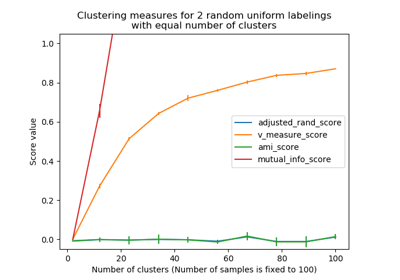# sklearn.metrics.mutual_info_score¶

sklearn.metrics.mutual_info_score(labels_true, labels_pred, contingency=None)[source]

Mutual Information between two clusterings.

The Mutual Information is a measure of the similarity between two labels of the same data. Where $$|U_i|$$ is the number of the samples in cluster $$U_i$$ and $$|V_j|$$ is the number of the samples in cluster $$V_j$$, the Mutual Information between clusterings $$U$$ and $$V$$ is given as:

$MI(U,V)=\sum_{i=1}^{|U|} \sum_{j=1}^{|V|} \frac{|U_i\cap V_j|}{N} \log\frac{N|U_i \cap V_j|}{|U_i||V_j|}$

This metric is independent of the absolute values of the labels: a permutation of the class or cluster label values won’t change the score value in any way.

This metric is furthermore symmetric: switching label_true with label_pred will return the same score value. This can be useful to measure the agreement of two independent label assignments strategies on the same dataset when the real ground truth is not known.

Read more in the User Guide.

Parameters
labels_trueint array, shape = [n_samples]

A clustering of the data into disjoint subsets.

labels_predint array-like of shape (n_samples,)

A clustering of the data into disjoint subsets.

contingency{None, array, sparse matrix}, shape = [n_classes_true, n_classes_pred]

A contingency matrix given by the contingency_matrix function. If value is None, it will be computed, otherwise the given value is used, with labels_true and labels_pred ignored.

Returns
mifloat

Mutual information, a non-negative value

adjusted_mutual_info_score

normalized_mutual_info_score
## Examples using sklearn.metrics.mutual_info_score¶